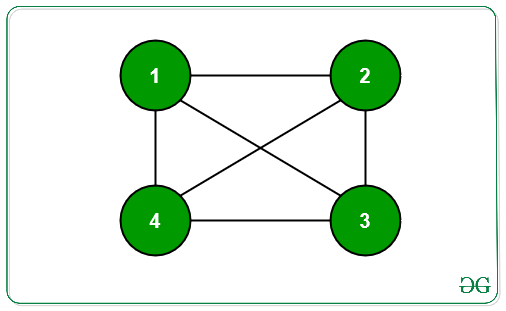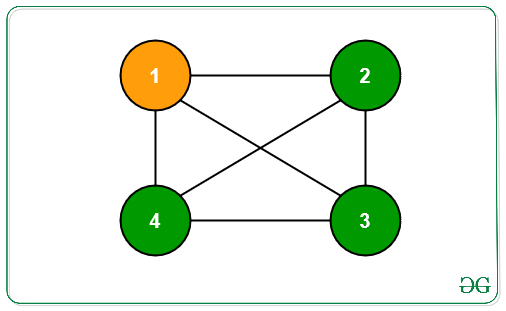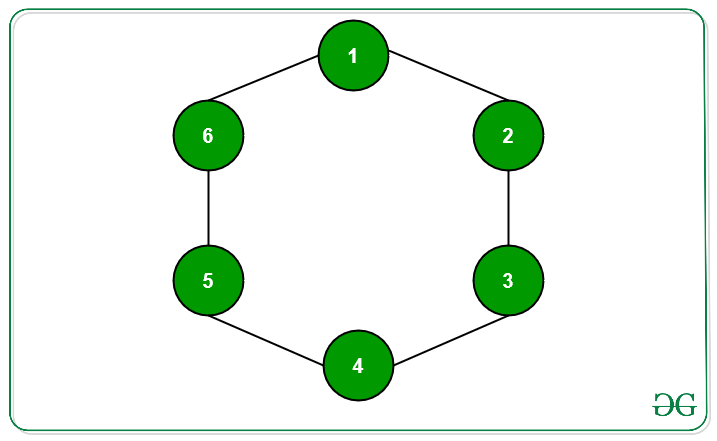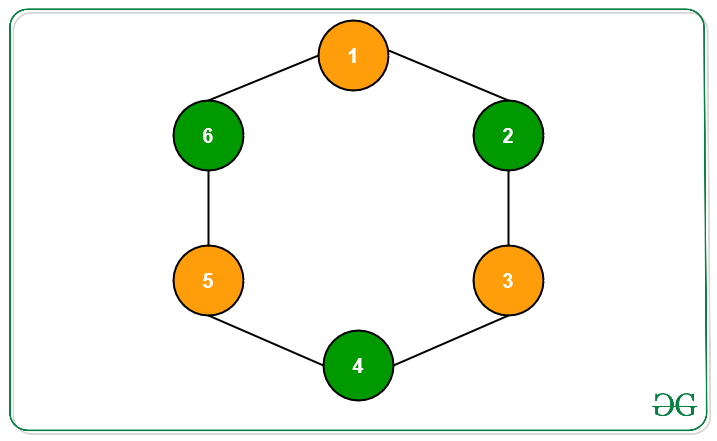Open in App
Not now

# Minimum nodes to be colored in a Graph such that every node has a colored neighbour

• Difficulty Level : Medium
• Last Updated : 23 Sep, 2021

Given a graph G with V nodes and E edges, the task is to colour no more than floor(V/2) nodes such that every node has at least one coloured node at a distance of atmost 1 unit. The distance between any two connected nodes of the graph is always exactly 1 unit. Print the nodes that need to be colored.

Examples:

Input: N = 4,
G:Output:Input: N = 6,
G:Output:Approach: This problem can be solved using BFS traversal. Follow the steps below to solve the problem:

• Initialize arrays odd[] and even[] to store nodes which are at a distance of odd and even number of nodes respectively from the source.
• Starting from the source node, perform BFS traversal with distance initialized 0, which denotes the distance from source node. Store all the nodes at a particular level in odd[] or even[] based on the value of distance.
• If distance is odd, that is (distance & 1) is 1, insert that node into odd[]. Otherwise, insert into even[].
• Now print the nodes from the array with minimum elements.
• Since minimum among count of nodes at odd distance or even distance does not exceed floor(V/2), the answer holds correct as every node at odd distance is connected to nodes at even distance and vice-versa.
• Hence, if number of nodes at even distance from source is less, print the nodes from even[]. Otherwise, print all nodes from odd[].

Illustration:
For the graph G given below,
Source Node S = 1• even[] = {1, 3, 5}
• odd[] = {2, 6, 4}
• minimum(even.size(), odd.size()) = min(3, 3) = 3
• Hence, coloring either all nodes at odd distance from the source or even distance from the source is correct as both their count is same.

Below is the implementation of the above approach:

## C++

 `// C++ Program to implement the` `// above approach`   `#include ` `using` `namespace` `std;`   `// Stores the graph` `map<``int``, vector<``int``> > graph;`   `// Stores the visited nodes` `map<``int``, ``int``> vis;`   `// Stores the nodes` `// at odd distance` `vector<``int``> odd;`   `// Stores the nodes at` `// even distance` `vector<``int``> even;`   `// Function to separate and` `// store the odd and even` `// distant nodes from source` `void` `bfs()` `{` `    ``// Source node` `    ``int` `src = 1;`   `    ``// Stores the nodes and their` `    ``// respective distances from` `    ``// the source` `    ``queue > q;`   `    ``// Insert the source` `    ``q.push({ src, 0 });`   `    ``// Mark the source visited` `    ``vis[src] = 1;`   `    ``while` `(!q.empty()) {`   `        ``// Extract a node from the` `        ``// front of the queue` `        ``int` `node = q.front().first;` `        ``int` `dist = q.front().second;` `        ``q.pop();`   `        ``// If distance from source` `        ``// is odd` `        ``if` `(dist & 1) {` `            ``odd.push_back(node);` `        ``}`   `        ``// Otherwise` `        ``else` `{` `            ``even.push_back(node);` `        ``}`   `        ``// Traverse its neighbors` `        ``for` `(``auto` `i : graph[node]) {`   `            ``// Insert its unvisited` `            ``// neighbours into the queue` `            ``if` `(!vis.count(i)) {`   `                ``q.push({ i, (dist + 1) });` `                ``vis[i] = 1;` `            ``}` `        ``}` `    ``}` `}`   `// Driver Program` `int` `main()` `{` `    ``graph.push_back(2);` `    ``graph.push_back(1);` `    ``graph.push_back(3);` `    ``graph.push_back(2);` `    ``graph.push_back(4);` `    ``graph.push_back(3);` `    ``graph.push_back(5);` `    ``graph.push_back(4);` `    ``graph.push_back(6);` `    ``graph.push_back(5);` `    ``graph.push_back(1);` `    ``graph.push_back(6);`   `    ``bfs();`   `    ``if` `(odd.size() < even.size()) {` `        ``for` `(``int` `i : odd) {` `            ``cout << i << ``" "``;` `        ``}` `    ``}` `    ``else` `{` `        ``for` `(``int` `i : even) {` `            ``cout << i << ``" "``;` `        ``}` `    ``}` `    ``return` `0;` `}`

## Java

 `// Java program to implement the` `// above approach` `import` `java.util.ArrayList;` `import` `java.util.HashMap;` `import` `java.util.LinkedList;` `import` `java.util.Map;` `import` `java.util.Queue;`   `class` `Pair ` `{` `    ``int` `first, second;`   `    ``public` `Pair(``int` `first, ``int` `second) ` `    ``{` `        ``this``.first = first;` `        ``this``.second = second;` `    ``}` `}`   `class` `GFG{`   `// Stores the graph` `static` `Map> graph = ``new` `HashMap<>();`   `// Stores the visited nodes` `static` `Map vis = ``new` `HashMap<>();`   `// Stores the nodes` `// at odd distance` `static` `ArrayList odd = ``new` `ArrayList<>();`   `// Stores the nodes at` `// even distance` `static` `ArrayList even = ``new` `ArrayList<>();`   `// Function to separate and` `// store the odd and even` `// distant nodes from source` `static` `void` `bfs()` `{` `    `  `    ``// Source node` `    ``int` `src = ``1``;`   `    ``// Stores the nodes and their` `    ``// respective distances from` `    ``// the source` `    ``Queue q = ``new` `LinkedList<>();`   `    ``// Insert the source` `    ``q.add(``new` `Pair(src, ``0``));`   `    ``// Mark the source visited` `    ``vis.put(src, ``1``);`   `    ``while` `(!q.isEmpty())` `    ``{` `        `  `        ``// Extract a node from the` `        ``// front of the queue` `        ``int` `node = q.peek().first;` `        ``int` `dist = q.peek().second;` `        ``q.poll();`   `        ``// If distance from source` `        ``// is odd` `        ``if` `((dist & ``1``) != ``0``) ` `        ``{` `            ``odd.add(node);` `        ``}`   `        ``// Otherwise` `        ``else` `        ``{` `            ``even.add(node);` `        ``}`   `        ``// Traverse its neighbors` `        ``for``(Integer i : graph.get(node)) ` `        ``{` `            `  `            ``// Insert its unvisited` `            ``// neighbours into the queue` `            ``if` `(!vis.containsKey(i))` `            ``{` `                ``q.add(``new` `Pair(i, (dist + ``1``)));` `                ``vis.put(i, ``1``);` `            ``}` `        ``}` `    ``}` `}`   `// Driver code` `public` `static` `void` `main(String[] args)` `{` `    ``graph.put(``1``, ``new` `ArrayList<>());` `    ``graph.put(``2``, ``new` `ArrayList<>());` `    ``graph.put(``3``, ``new` `ArrayList<>());` `    ``graph.put(``4``, ``new` `ArrayList<>());` `    ``graph.put(``5``, ``new` `ArrayList<>());` `    ``graph.put(``6``, ``new` `ArrayList<>());` `    ``graph.get(``1``).add(``2``);` `    ``graph.get(``2``).add(``1``);` `    ``graph.get(``2``).add(``3``);` `    ``graph.get(``3``).add(``2``);` `    ``graph.get(``3``).add(``4``);` `    ``graph.get(``4``).add(``3``);` `    ``graph.get(``4``).add(``5``);` `    ``graph.get(``5``).add(``4``);` `    ``graph.get(``5``).add(``6``);` `    ``graph.get(``6``).add(``5``);` `    ``graph.get(``6``).add(``1``);` `    ``graph.get(``1``).add(``6``);`   `    ``bfs();`   `    ``if` `(odd.size() < even.size()) ` `    ``{` `        ``for``(``int` `i : odd)` `        ``{` `            ``System.out.print(i + ``" "``);` `        ``}` `    ``} ` `    ``else` `    ``{` `        ``for``(``int` `i : even)` `        ``{` `            ``System.out.print(i + ``" "``);` `        ``}` `    ``}` `}` `}`   `// This code is contributed by sanjeev2552`

## Python3

 `# Python3 Program to implement the` `# above approach` ` `  `# Stores the graph` `graph ``=` `dict``()` ` `  `# Stores the visited nodes` `vis ``=` `dict``()` ` `  `# Stores the nodes` `# at odd distance` `odd ``=` `[]` ` `  `# Stores the nodes at` `# even distance` `even ``=` `[]` ` `  `# Function to separate and` `# store the odd and even` `# distant nodes from source` `def` `bfs():`   `    ``# Source node` `    ``src ``=` `1``;` ` `  `    ``# Stores the nodes and their` `    ``# respective distances from` `    ``# the source` `    ``q ``=` `[]` ` `  `    ``# Insert the source` `    ``q.append([ src, ``0` `]);` ` `  `    ``# Mark the source visited` `    ``vis[src] ``=` `1``;` ` `  `    ``while` `(``len``(q) !``=` `0``):` ` `  `        ``# Extract a node from the` `        ``# front of the queue` `        ``node ``=` `q[``0``][``0``]` `        ``dist ``=` `q[``0``][``1``]` `        ``q.pop(``0``);` ` `  `        ``# If distance from source` `        ``# is odd` `        ``if` `(dist & ``1``):` `            ``odd.append(node);` ` `  `        ``# Otherwise` `        ``else``:` `            ``even.append(node);` `         `  `        ``# Traverse its neighbors` `        ``for` `i ``in` `graph[node]:` ` `  `            ``# Insert its unvisited` `            ``# neighbours into the queue` `            ``if` `(i ``not` `in` `vis):` ` `  `                ``q.append([ i, (dist ``+` `1``) ]);` `                ``vis[i] ``=` `1``;` `             `  `# Driver code` `if` `__name__``=``=``'__main__'``:`   `    ``graph[``1``] ``=` `[``2``, ``6``]` `    ``graph[``2``] ``=` `[``1``, ``3``]` `    ``graph[``3``] ``=` `[``2``, ``4``]` `    ``graph[``4``] ``=` `[``3``, ``5``]` `    ``graph[``5``] ``=` `[``4``, ``6``]` `    ``graph[``6``] ``=` `[``5``, ``1``]` ` `  `    ``bfs();` ` `  `    ``if` `(``len``(odd) < ``len``(even)):` `        ``for` `i ``in` `odd:` `            ``print``(i, end ``=` `' '``)` `            `  `    ``else``:` `        ``for` `i ``in` `even:` `            ``print``(i, end ``=` `' '``)` `            `  `# This code is contributed by rutvik_56`

Output:

`1 3 5`

Time Complexity: O(V + E)
Auxiliary Space: O(V)

My Personal Notes arrow_drop_up
Related Articles